[TOC]

# Overview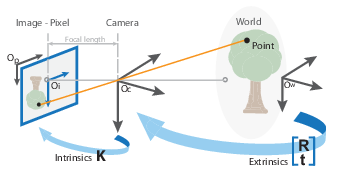## Lens Projections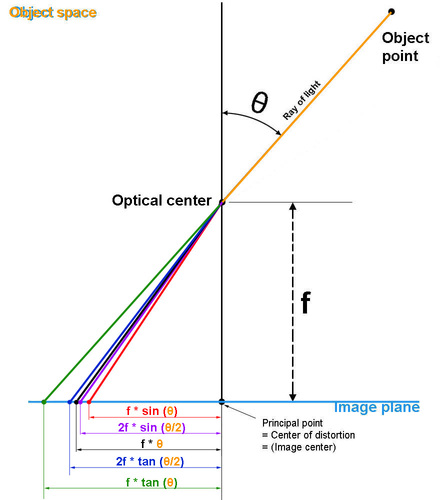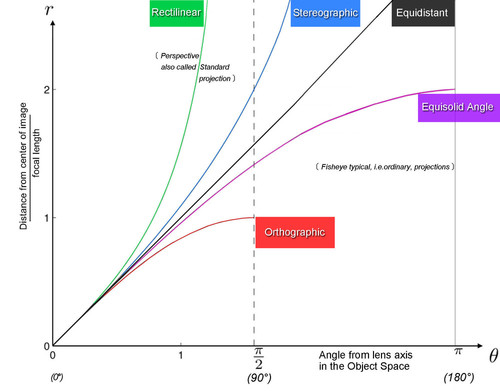• Perspective and fisheye imaging process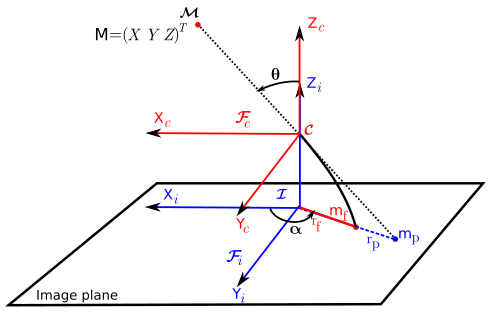## Optics: Terminology

• Dioptric: All elements are refractive (lenses)
• Catoptric: All elements are reflective (mirrors)
• Catadioptric: Elements are refractive and reflective (mirrors + lenses)

# Pinhole Camera

• pinhole model (rectilinear projection model) + radial-tangential distortion

$K = \left[\begin{array}{ccc} f_x & 0 & c_x \\ 0 & f_y & c_y \\ 0 & 0 & 1 \end{array}\right]$

$D = \left[ k_1, k_2, p_1, p_2 \right]$

The Pinhole camera model is the most common camera model for consumer cameras. In this model, the image is mapped onto a plane through perspective projection. The projection is defined by the camera intrinsic parameters such as focal length, principal point, aspect ratio, and skew.

# Fisheye / Omnidirectional Camera 

## OpenCV (Equidistant / KannalaBrandt) fisheye camera model 😄• pinhole model (rectilinear projection model) + fisheye distortion

$K = \left[\begin{array}{ccc} f_x & 0 & c_x \\ 0 & f_y & c_y \\ 0 & 0 & 1 \end{array}\right]$

$D = \left[ k_1, k_2, k_3, k_4 \right]$

The Fisheye camera model is a camera model utilized for wide field of view cameras. This camera model is neccessary because the pinhole perspective camera model is not capable of modeling image projections as the field of view approaches 180 degrees.

### Pinhole (Rectilinear) Projection

$r = f \cdot \tan(\theta)$

Given a point $X=[x_c y_c z_c]$ from the camera $$z_c=1$$ plane in camera coordinates, the pinhole projection is:

$\begin{cases} r = \sqrt{x_c^2 + y_c^2} \\ \theta = \arctan(r, |z_c|) = \arctan(r, 1) = \arctan(r) \end{cases}$

### Fisheye (Equidistant) Projection

$r = f \cdot \theta$

fisheye distortion:

$\theta_d = \theta (1 + k1 \cdot \theta^2 + k2 \cdot \theta^4 + k3 \cdot \theta^6 + k4 \cdot \theta^8)$

The distorted point coordinates are

$\begin{cases} x_d = \frac{\theta_d}{r} \cdot x_c \\ y_d = \frac{\theta_d}{r} \cdot y_c \end{cases}$

convert into pixel coordinates, the final pixel coordinates vector (assume $$\alpha=0$$)

$\begin{cases} u = f_x \cdot x_d + c_x \\ v = f_y \cdot y_d + c_y \end{cases}$

write in matrix form

\begin{aligned} \left[\begin{array}{c}u\\v\\1\end{array}\right] = \left[\begin{array}{ccc} f_x & 0 & c_x \\ 0 & f_y & c_y \\ 0 & 0 & 1 \end{array}\right] \left[\begin{array}{c}x_d\\y_d\\1\end{array}\right] \end{aligned}

in ORB-SLAM3:

## ATAN model

• pinhole model (rectilinear projection model) + FOV distortion

$K = \left[\begin{array}{ccc} f_x & 0 & c_x \\ 0 & f_y & c_y \\ 0 & 0 & 1 \end{array}\right]$

$D = \left[ \omega \right]$

Used in

• PTAM 
• LSD-SLAM

This is an alternative representation for camera models with large radial distortion (such as fisheye cameras) where the distance between an image point and principal point is roughly proportional to the angle between the 3D point and the optical axis. This camera model is first proposed in Straight Lines Have to be Straight: Automatic Calibration and Removal of Distortion from Scenes of Structured Environments.

Given a point $X=[x_c y_c z_c]$ from the camera $$z_c=1$$ plane in camera coordinates, the pinhole projection is:

$r = \sqrt{\frac{x_c^2 + y_c^2}{z_c^2}} = \sqrt{x_c^2 + y_c^2}$

FOV distortion:

$r_d = \frac{1}{\omega} \arctan( 2 \cdot r \cdot \tan(\frac{\omega}{2}) )$

where $$\omega$$ is the FOV distortion coefficient

The distorted point coordinates are

$\begin{cases} x_d = \frac{r_d}{r} \cdot x_c \\ y_d = \frac{r_d}{r} \cdot y_c \end{cases}$

convert into pixel coordinates, the final pixel coordinates vector

\begin{aligned} \left[\begin{array}{c}u\\v\\1\end{array}\right] = \left[\begin{array}{ccc} f_x & 0 & c_x \\ 0 & f_y & c_y \\ 0 & 0 & 1 \end{array}\right] \left[\begin{array}{c}x_d\\y_d\\1\end{array}\right] \end{aligned}

in PTAM

## MEI (Unified Camera Model)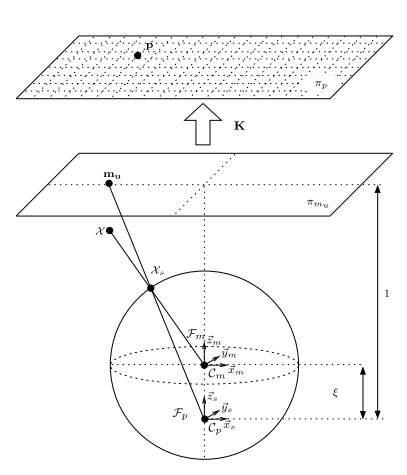## PolyFisheye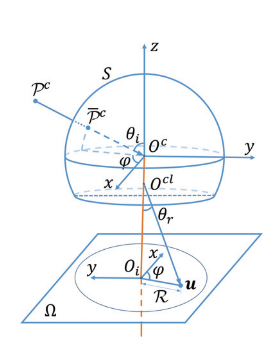## EUCM 😄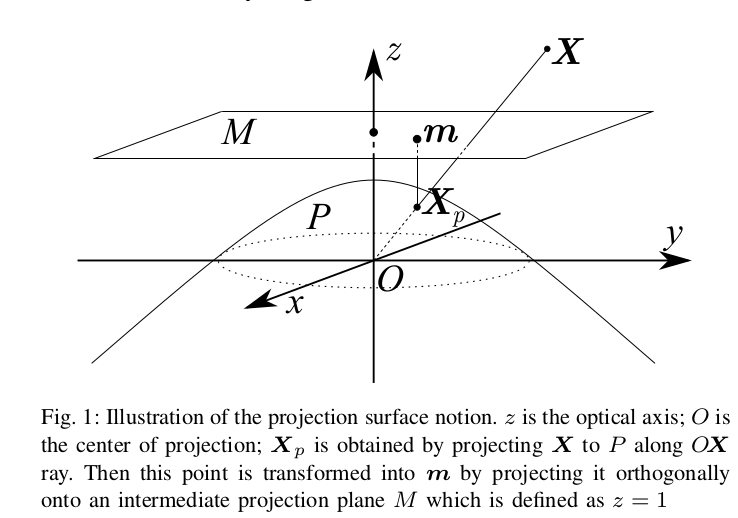• 相机坐标系： 真实世界坐标中的位置坐标，单位为m，一般对应在表达为Ｘ

• 椭球面坐标系：是一个中转球面，与对应点的世界坐标相差一个scale的系数；也成为Ｐ平面，对应表达为Xp

• Z=1平面：是椭球面上的点在z=1平面上的投影，也称为M平面，其x，y值与Xp一样，只是z值固定为1，对应表达为m, 也是归一化平面

• 像素坐标系： 图片中点像素点坐标，左上角为(0,0)，由 M平面 中的点经过内参矩阵K计算而来；对应表达为p(u,v)

# Models in Code

• Supported models in Kalibr

• Distortion Models in ROS (distortion_models.h)

• plumb_bob: a 5-parameter polynomial approximation of radial and tangential distortion
• rational_polynomial: an 8-parameter rational polynomial distortion model
• equidistant (lunar)
• PTAM  : support ATAN camera model

• uzh-rpg/rpg_vikit/vikit_common: support pinhole, atan and omni camera

• ethz-asl/aslam_cv2: support pinhole and unified peojection and radtan, fisheye and equidistant distortion

• cggos/okvis_cg: support pinhole peojection and radtan and equidistant distortion

• VINS-Mono: Pinhole, Fisheye (Kannala-Brandt, MEI)

• hengli/camodocal
• Pinhole camera model
• Unified projection model
• Equidistant fish-eye model
• OpenVINS: Pinhole, Fisheye (Kannala-Brandt)

• ORB-SLAM3: Pinhole, Fisheye (Kannala-Brandt)

# Impact of Model Changes on vSLAM

Eg. Pinhole --> EUCM，将去畸变坐标变成了椭球面坐标

• 投影和反投影

• Pinhole: 像素坐标系 to 归一化平面坐标系
• EUCM: 像素坐标系 to 椭球面坐标系
• 三角化

• Pinhole: 去畸变 归一化平面坐标系
• EUCM: 椭球面坐标系
• 计算基本矩阵和本质矩阵

• 优化 (残差 或 雅可比矩阵)

• Pinhole: 去畸变 像素坐标系 或 归一化平面坐标系
• EUCM: 像素坐标系 或 椭球面坐标系 (或 平面坐标系)

# Papers

• Straight Lines Have to be Straight: Automatic Calibration and Removal of Distortion from Scenes of Structured Environments

• A Generic Camera Model and Calibration Method for Conventional, Wide-Angle, and Fish-Eye Lenses

• Single View Point Omnidirectional Camera Calibration from Planar Grids

• The Double Sphere Camera Model 

# Reference

Camera Models Summary
https://cgabc.xyz/posts/c60702bf/

Gavin Gao

2019年1月26日